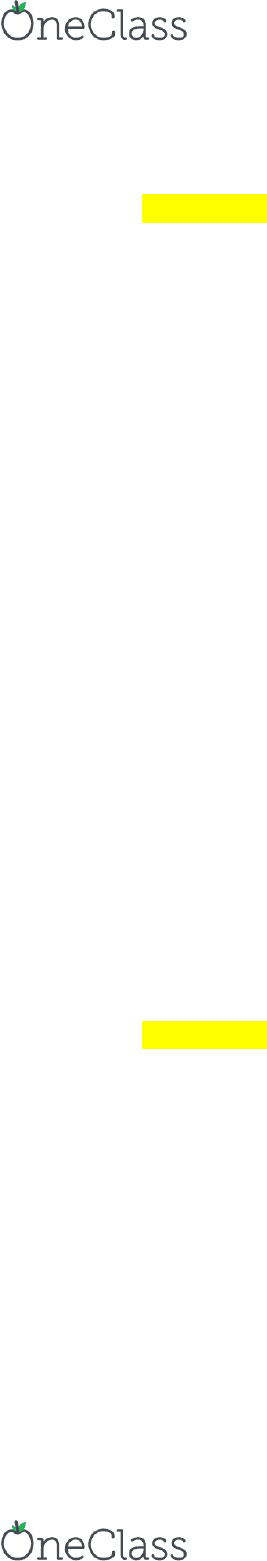# FIN 3410 Lecture Notes - Lecture 13: Arbitrage

14 views3 pages
School
Department
Course

For unlimited access to Class Notes, a Class+ subscription is required.Day 13 & 14 Notes
EX CH15 5
Background
Currency carry trade
A Bermuda-based hedge fund manager
I\$ = 6%
IY = 2.8%
The fund manager borrowed Y 1 billion for one year and invested in the US
So = Y95/\$
S1 = Y105/\$
Problem
Profit(\$) = revenue(\$) cost(\$)
o Revenue(\$) = FV(\$investment)
o Cost(\$) = \$equivalent of Y(loan+int)
1. Revenue(\$) = FV (\$ investment)
a. = [loanY / So(Y/\$)] * (1 + i\$)
b. = [Y1 billion / (Y95/\$)] * (1 + 0.06)
c. = \$11,157,895
2. Cost(\$) = \$equivalent of Y(loan+int)
a. = Y(loan+int) / S1(Y/\$)
b. = [Y1 billion * (1 + 0.028)] / (Y105/\$)
c. = \$9,790,476
3. Profit(\$) = revenue(\$) cost(\$)
a. = \$11,157,895 - \$9,790,476
b. = \$1,367,419
i. A loss is okay
EX CH15 4
Background
Io\$ = \$10,000,000 initial investment in pure-discount bonds
So = Y 80/\$
I1\$ = \$10,650,000
S1 = Y 110/\$
rY = ?
S1 > So
o \$ appreciation against Y
Approach 1
I1Y = I1\$ * S1(Y/\$)
o \$10,650,000 * Y 110/\$ = Y 1,171,500,000
find more resources at oneclass.com
find more resources at oneclass.com
Unlock document

This preview shows page 1 of the document.
Unlock all 3 pages and 3 million more documents.

Already have an account? Log in

# Get access

Grade+
\$10 USD/m
Billed \$120 USD annually
Homework Help
Class Notes
Textbook Notes
40 Verified Answers
Study Guides
1 Booster Class
Class+
\$8 USD/m
Billed \$96 USD annually
Homework Help
Class Notes
Textbook Notes
30 Verified Answers
Study Guides
1 Booster Class